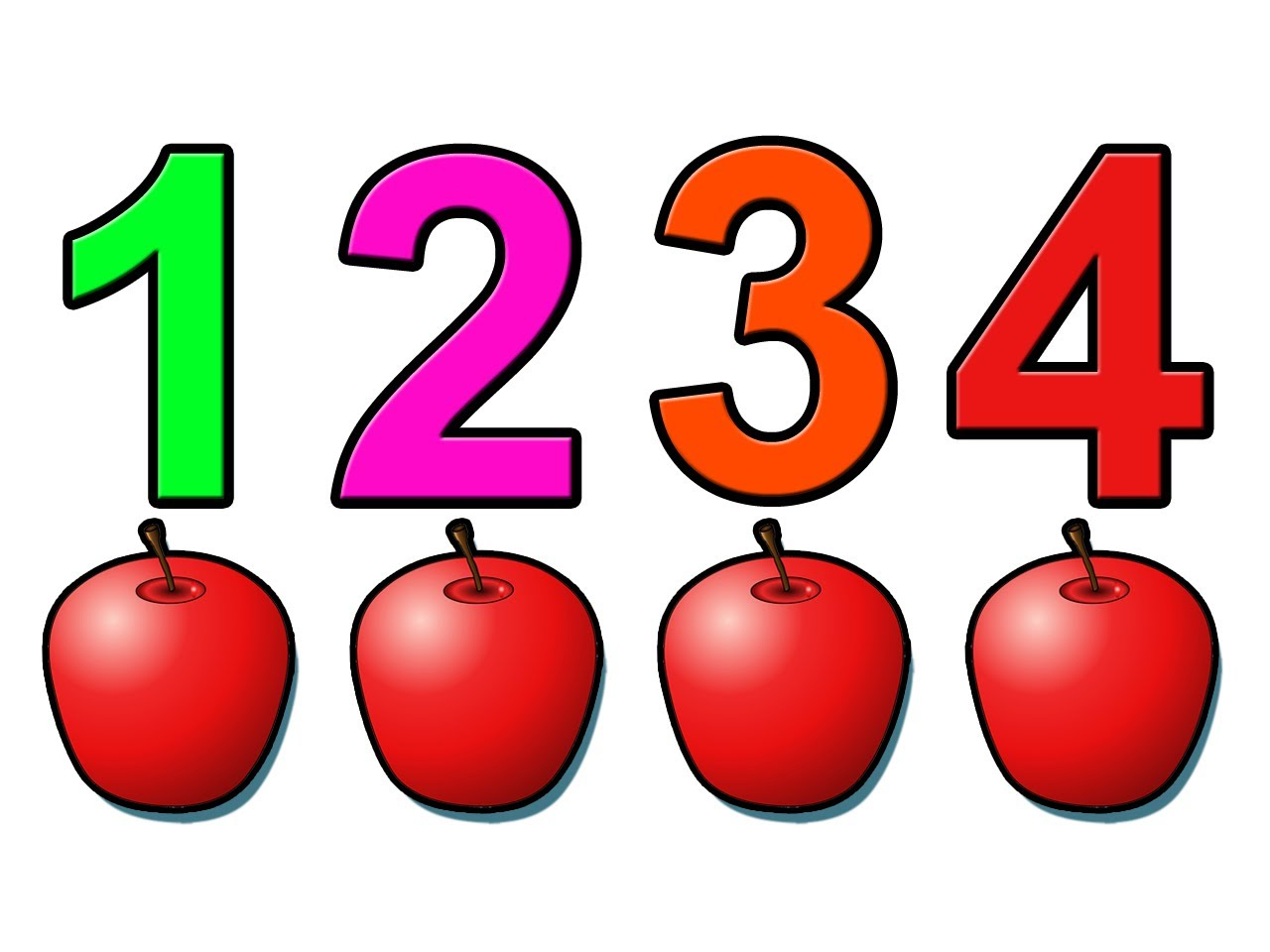# 1234 Followers. A problem for "1234"

Probability Level 5If the total number of $8$-digit positive integers that can be formed using the digits $0$ to $9$ inclusive and without repetition. such that $\overline{1234}$ always exist collectively in the same order in the positive integer is $P$.

We coin the term: Cute divisors. A cute divisor of a number $n$ is a positive integer other than $1$ and $n$ which divide the number $n$ completely and leaves no remainder.

Find the sum of all cute divisors of $P$.

×